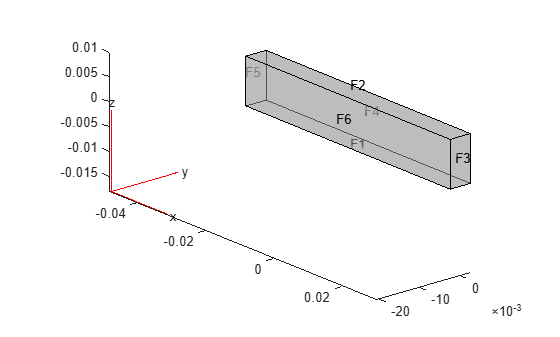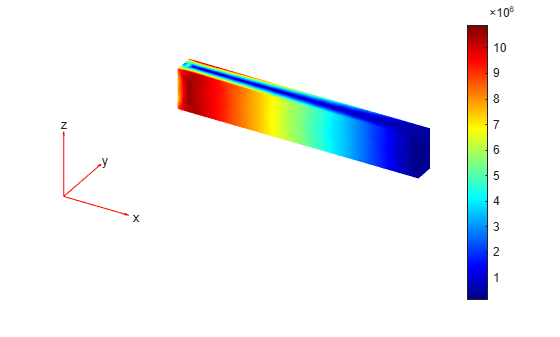evaluatePrincipalStress

Evaluate principal stress at nodal locations

Description

example

pStress = evaluatePrincipalStress(structuralresults) evaluates principal stress at nodal locations using stress values from structuralresults. For transient and frequency response structural models, evaluatePrincipalStress evaluates principal stress for all time- and frequency-steps, respectively.

Examples

collapse all

Solve a static structural model representing a bimetallic cable under tension, and compute octahedral shear stress.

Create a structural model.

structuralmodel = createpde('structural','static-solid');

Create a geometry and include it in the model. Plot the geometry.

gm = multicylinder([0.01,0.015],0.05);
structuralmodel.Geometry = gm;
pdegplot(structuralmodel,'FaceLabels','on', ...
'CellLabels','on', ...
'FaceAlpha',0.5)Specify the Young's modulus and Poisson's ratio for each metal.

structuralProperties(structuralmodel,'Cell',1,'YoungsModulus',110E9, ...
'PoissonsRatio',0.28);
structuralProperties(structuralmodel,'Cell',2,'YoungsModulus',210E9, ...
'PoissonsRatio',0.3);

Specify that faces 1 and 4 are fixed boundaries.

structuralBC(structuralmodel,'Face',[1,4],'Constraint','fixed');

Specify the surface traction for faces 2 and 5.

'SurfaceTraction',[0;0;100]);

Generate a mesh and solve the problem.

generateMesh(structuralmodel);
structuralresults = solve(structuralmodel)
structuralresults =
StaticStructuralResults with properties:

Displacement: [1x1 FEStruct]
Strain: [1x1 FEStruct]
Stress: [1x1 FEStruct]
VonMisesStress: [22281x1 double]
Mesh: [1x1 FEMesh]

Evaluate the principal stress at nodal locations.

pStress = evaluatePrincipalStress(structuralresults);

Use the principal stress to evaluate the first and second invariant of stress.

I1 = pStress.s1 + pStress.s2 + pStress.s3;
I2 = pStress.s1.*pStress.s2 + ...
pStress.s2.*pStress.s3 + ...
pStress.s3.*pStress.s1;
tauOct = sqrt(2*(I1.^2 -3*I2))/3;
pdeplot3D(structuralmodel,'ColorMapData',tauOct)Evaluate the principal stress and octahedral shear stress in a beam under a harmonic excitation.

Create a transient dynamic model for a 3-D problem.

structuralmodel = createpde('structural','transient-solid');

Create the geometry and include it in the model. Plot the geometry.

gm = multicuboid(0.06,0.005,0.01);
structuralmodel.Geometry = gm;
pdegplot(structuralmodel,'FaceLabels','on','FaceAlpha',0.5)
view(50,20)Specify the Young's modulus, Poisson's ratio, and mass density of the material.

structuralProperties(structuralmodel,'YoungsModulus',210E9, ...
'PoissonsRatio',0.3, ...
'MassDensity',7800);

Fix one end of the beam.

structuralBC(structuralmodel,'Face',5,'Constraint','fixed');

Apply a sinusoidal displacement along the y-direction on the end opposite the fixed end of the beam.

structuralBC(structuralmodel,'Face',3,...
'YDisplacement',1E-4,...
'Frequency',50);

Generate a mesh.

generateMesh(structuralmodel,'Hmax',0.01);

Specify the zero initial displacement and velocity.

structuralIC(structuralmodel,'Displacement',[0;0;0],'Velocity',[0;0;0]);

Solve the model.

tlist = 0:0.002:0.2;
structuralresults = solve(structuralmodel,tlist);

Evaluate the principal stress in the beam.

pStress = evaluatePrincipalStress(structuralresults);

Use the principal stress to evaluate the first and second invariants.

I1 = pStress.s1 + pStress.s2 + pStress.s3;
I2 = pStress.s1.*pStress.s2 + ...
pStress.s2.*pStress.s3 + ...
pStress.s3.*pStress.s1;

Use the stress invariants to compute the octahedral shear stress.

tauOct = sqrt(2*(I1.^2 -3*I2))/3;

Plot the results.

figure
pdeplot3D(structuralmodel,'ColorMapData',tauOct(:,end))Input Arguments

collapse all

Solution of the structural analysis problem, specified as a StaticStructuralResults, TransientStructuralResults, or FrequencyStructuralResults object. Create structuralresults by using the solve function.

Example: structuralresults = solve(structuralmodel)

Output Arguments

collapse all

Principal stress at the nodal locations, returned as a structure array.

Introduced in R2017b

Partial Differential Equation Toolbox DocumentationGet trial now